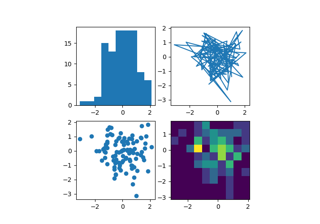# matplotlib.pyplot.loglog¶

matplotlib.pyplot.loglog(*args, **kwargs)[source]

Make a plot with log scaling on both the x and y axis.

Call signatures:

loglog([x], y, [fmt], data=None, **kwargs)
loglog([x], y, [fmt], [x2], y2, [fmt2], ..., **kwargs)


This is just a thin wrapper around plot which additionally changes both the x-axis and the y-axis to log scaling. All of the concepts and parameters of plot can be used here as well.

The additional parameters base, subs and nonpositive control the x/y-axis properties. They are just forwarded to Axes.set_xscale and Axes.set_yscale. To use different properties on the x-axis and the y-axis, use e.g. ax.set_xscale("log", base=10); ax.set_yscale("log", base=2).

Parameters: basefloat, default: 10Base of the logarithm. subssequence, optionalThe location of the minor ticks. If None, reasonable locations are automatically chosen depending on the number of decades in the plot. See Axes.set_xscale/Axes.set_yscale for details. nonpositive{'mask', 'clip'}, default: 'mask'Non-positive values can be masked as invalid, or clipped to a very small positive number. linesA list of Line2D objects representing the plotted data. **kwargsAll parameters supported by plot.

## Examples using matplotlib.pyplot.loglog¶